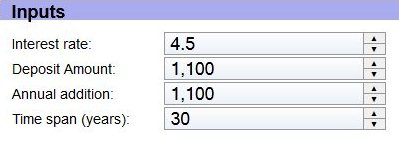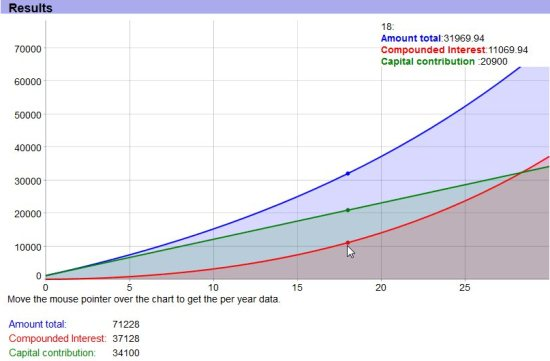Editor Ratings:
User Ratings:
[Total: 0 Average: 0]

Online compound interest calculator is a free online calculator to calculate compound interest. Visit the website and calculate the compound interest for your desired principal amount.

Just provide the starting amount, interest rate, annual addition, and span in years. This will then calculate the interest that you accumulate over the span of your deposit.Compound Interest sums up when you add your interest to the principal amount. This addition of interest to principal amount is called compounding. The interest rate is the annual interest rate of your investment. The initial investment or deposit amount is known as principal amount. You should also know the number of years you are going to invest. If you know all these values then you can calculate your compound interest with the help of this website.

The formula for calculating compound interest is P=C(1+r/n)^nt

Where P= Future value

C=Initial deposit

r=Interest Rate

n=number of times per year interest is compounded

t=number of years investedJust input the values of: Interest Rate, Deposit Amount, Annual Addition, and Time Span; as shown in above screenshot. You can directly enter the values or just click on the arrows next to them to increase or decrease the corresponding values. After you enter your values, this calculator shows a nice graph; that shows how does your total amount changes over the span of deposit. As you keep changing the values, the graph also keeps changing. The graph is shown in below screenshot.The results are also interpreted below the graph in a numerical form in terms of: Amount Total, Compound Interest, and Capital Contribution. If you want to see the values at a particular point, then just move your mouse over the chart to get the data per year. The definitions of the terms are also given in the website.

This Online compound interest calculator is completely free. Try it here. Also check out online Amortization schedule calculator to calculate amortization amount and loan summary online.